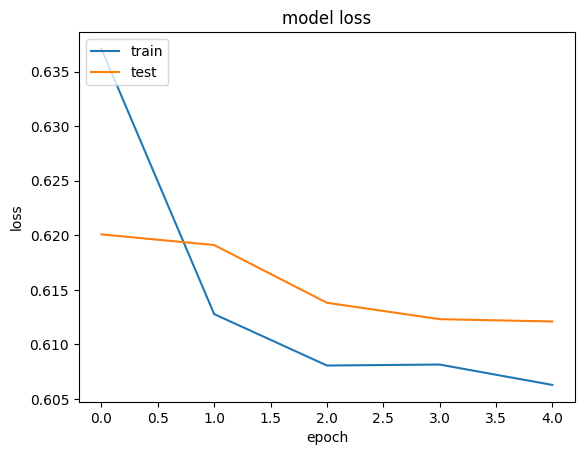» Code examples / Structured Data / Collaborative Filtering for Movie Recommendations

# Collaborative Filtering for Movie Recommendations

Author: Siddhartha Banerjee
Date created: 2020/05/24
Description: Recommending movies using a model trained on Movielens dataset.View in ColabGitHub source

## Introduction

This example demonstrates Collaborative filtering using the Movielens dataset to recommend movies to users. The MovieLens ratings dataset lists the ratings given by a set of users to a set of movies. Our goal is to be able to predict ratings for movies a user has not yet watched. The movies with the highest predicted ratings can then be recommended to the user.

The steps in the model are as follows:

1. Map user ID to a "user vector" via an embedding matrix
2. Map movie ID to a "movie vector" via an embedding matrix
3. Compute the dot product between the user vector and movie vector, to obtain the a match score between the user and the movie (predicted rating).
4. Train the embeddings via gradient descent using all known user-movie pairs.

References:

``````import pandas as pd
import numpy as np
from zipfile import ZipFile
import tensorflow as tf
from tensorflow import keras
from tensorflow.keras import layers
from pathlib import Path
import matplotlib.pyplot as plt
``````

## First, load the data and apply preprocessing

``````# Download the actual data from http://files.grouplens.org/datasets/movielens/ml-latest-small.zip"
# Use the ratings.csv file
movielens_data_file_url = (
"http://files.grouplens.org/datasets/movielens/ml-latest-small.zip"
)
movielens_zipped_file = keras.utils.get_file(
"ml-latest-small.zip", movielens_data_file_url, extract=False
)
keras_datasets_path = Path(movielens_zipped_file).parents
movielens_dir = keras_datasets_path / "ml-latest-small"

# Only extract the data the first time the script is run.
if not movielens_dir.exists():
with ZipFile(movielens_zipped_file, "r") as zip:
# Extract files
print("Extracting all the files now...")
zip.extractall(path=keras_datasets_path)
print("Done!")

ratings_file = movielens_dir / "ratings.csv"
``````

First, need to perform some preprocessing to encode users and movies as integer indices.

``````user_ids = df["userId"].unique().tolist()
user2user_encoded = {x: i for i, x in enumerate(user_ids)}
userencoded2user = {i: x for i, x in enumerate(user_ids)}
movie_ids = df["movieId"].unique().tolist()
movie2movie_encoded = {x: i for i, x in enumerate(movie_ids)}
movie_encoded2movie = {i: x for i, x in enumerate(movie_ids)}
df["user"] = df["userId"].map(user2user_encoded)
df["movie"] = df["movieId"].map(movie2movie_encoded)

num_users = len(user2user_encoded)
num_movies = len(movie_encoded2movie)
df["rating"] = df["rating"].values.astype(np.float32)
# min and max ratings will be used to normalize the ratings later
min_rating = min(df["rating"])
max_rating = max(df["rating"])

print(
"Number of users: {}, Number of Movies: {}, Min rating: {}, Max rating: {}".format(
num_users, num_movies, min_rating, max_rating
)
)
``````
``````Number of users: 610, Number of Movies: 9724, Min rating: 0.5, Max rating: 5.0
``````

## Prepare training and validation data

``````df = df.sample(frac=1, random_state=42)
x = df[["user", "movie"]].values
# Normalize the targets between 0 and 1. Makes it easy to train.
y = df["rating"].apply(lambda x: (x - min_rating) / (max_rating - min_rating)).values
# Assuming training on 90% of the data and validating on 10%.
train_indices = int(0.9 * df.shape)
x_train, x_val, y_train, y_val = (
x[:train_indices],
x[train_indices:],
y[:train_indices],
y[train_indices:],
)
``````

## Create the model

We embed both users and movies in to 50-dimensional vectors.

The model computes a match score between user and movie embeddings via a dot product, and adds a per-movie and per-user bias. The match score is scaled to the `[0, 1]` interval via a sigmoid (since our ratings are normalized to this range).

``````EMBEDDING_SIZE = 50

class RecommenderNet(keras.Model):
def __init__(self, num_users, num_movies, embedding_size, **kwargs):
super(RecommenderNet, self).__init__(**kwargs)
self.num_users = num_users
self.num_movies = num_movies
self.embedding_size = embedding_size
self.user_embedding = layers.Embedding(
num_users,
embedding_size,
embeddings_initializer="he_normal",
embeddings_regularizer=keras.regularizers.l2(1e-6),
)
self.user_bias = layers.Embedding(num_users, 1)
self.movie_embedding = layers.Embedding(
num_movies,
embedding_size,
embeddings_initializer="he_normal",
embeddings_regularizer=keras.regularizers.l2(1e-6),
)
self.movie_bias = layers.Embedding(num_movies, 1)

def call(self, inputs):
user_vector = self.user_embedding(inputs[:, 0])
user_bias = self.user_bias(inputs[:, 0])
movie_vector = self.movie_embedding(inputs[:, 1])
movie_bias = self.movie_bias(inputs[:, 1])
dot_user_movie = tf.tensordot(user_vector, movie_vector, 2)
# Add all the components (including bias)
x = dot_user_movie + user_bias + movie_bias
# The sigmoid activation forces the rating to between 0 and 1
return tf.nn.sigmoid(x)

model = RecommenderNet(num_users, num_movies, EMBEDDING_SIZE)
model.compile(
)
``````

## Train the model based on the data split

``````history = model.fit(
x=x_train,
y=y_train,
batch_size=64,
epochs=5,
verbose=1,
validation_data=(x_val, y_val),
)
``````
``````Epoch 1/5
1418/1418 [==============================] - 6s 4ms/step - loss: 0.6368 - val_loss: 0.6206
Epoch 2/5
1418/1418 [==============================] - 7s 5ms/step - loss: 0.6131 - val_loss: 0.6176
Epoch 3/5
1418/1418 [==============================] - 6s 4ms/step - loss: 0.6083 - val_loss: 0.6146
Epoch 4/5
1418/1418 [==============================] - 6s 4ms/step - loss: 0.6072 - val_loss: 0.6131
Epoch 5/5
1418/1418 [==============================] - 6s 4ms/step - loss: 0.6075 - val_loss: 0.6150
``````

## Plot training and validation loss

``````plt.plot(history.history["loss"])
plt.plot(history.history["val_loss"])
plt.title("model loss")
plt.ylabel("loss")
plt.xlabel("epoch")
plt.legend(["train", "test"], loc="upper left")
plt.show()
``````## Show top 10 movie recommendations to a user

``````movie_df = pd.read_csv(movielens_dir / "movies.csv")

# Let us get a user and see the top recommendations.
user_id = df.userId.sample(1).iloc
movies_watched_by_user = df[df.userId == user_id]
movies_not_watched = movie_df[
~movie_df["movieId"].isin(movies_watched_by_user.movieId.values)
]["movieId"]
movies_not_watched = list(
set(movies_not_watched).intersection(set(movie2movie_encoded.keys()))
)
movies_not_watched = [[movie2movie_encoded.get(x)] for x in movies_not_watched]
user_encoder = user2user_encoded.get(user_id)
user_movie_array = np.hstack(
([[user_encoder]] * len(movies_not_watched), movies_not_watched)
)
ratings = model.predict(user_movie_array).flatten()
top_ratings_indices = ratings.argsort()[-10:][::-1]
recommended_movie_ids = [
movie_encoded2movie.get(movies_not_watched[x]) for x in top_ratings_indices
]

print("Showing recommendations for user: {}".format(user_id))
print("====" * 9)
print("Movies with high ratings from user")
print("----" * 8)
top_movies_user = (
movies_watched_by_user.sort_values(by="rating", ascending=False)
.movieId.values
)
movie_df_rows = movie_df[movie_df["movieId"].isin(top_movies_user)]
for row in movie_df_rows.itertuples():
print(row.title, ":", row.genres)

print("----" * 8)
print("Top 10 movie recommendations")
print("----" * 8)
recommended_movies = movie_df[movie_df["movieId"].isin(recommended_movie_ids)]
for row in recommended_movies.itertuples():
print(row.title, ":", row.genres)
``````
``````Showing recommendations for user: 474
====================================
Movies with high ratings from user
--------------------------------
Fugitive, The (1993) : Thriller
Remains of the Day, The (1993) : Drama|Romance
West Side Story (1961) : Drama|Musical|Romance
X2: X-Men United (2003) : Action|Adventure|Sci-Fi|Thriller
--------------------------------
Top 10 movie recommendations
--------------------------------
Dazed and Confused (1993) : Comedy
Ghost in the Shell (Kôkaku kidôtai) (1995) : Animation|Sci-Fi
Drugstore Cowboy (1989) : Crime|Drama